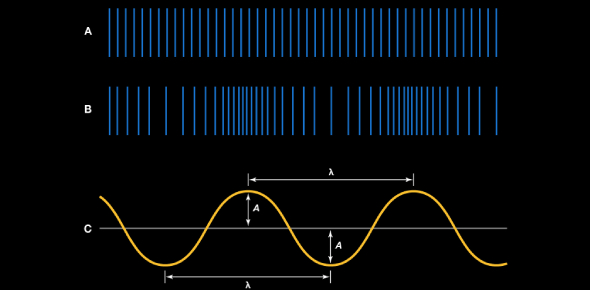# Types Of Sound Waves! Trivia Questions Quiz

63 Questions | Total Attempts: 66Settings.

• 1.
How and why the brain interprets sound?
• A.

Psychoacoustics

• B.

Sound pressure waves

• C.

Refraction

• D.

Phase

• E.

Hertz

• 2.
Atmospheric pressure is called?
• A.

Psychoacoustics

• B.

Sound pressure waves

• C.

Refraction

• D.

Phase

• E.

Hertz

• 3.
When dealing with sound waves, compression is?
• A.

Greater than normal atmospheric pressure.

• B.

Less than normal atmospheric pressure.

• C.

Pushing against lower pressure waves. ( in and outward direction)

• D.

Pushing against higher pressure waves. ( out and inward direction)

• 4.
When dealing with sound waves, rarefaction is?
• A.

Greater than normal atmospheric pressure.

• B.

Less than normal atmospheric pressure.

• C.

Pushing against lower pressure waves. ( in and outward direction)

• D.

Pushing against higher pressure waves. ( out and inward direction)

• 5.
When dealing with sound waves, wave propagation is?
• A.

Greater than normal atmospheric pressure.

• B.

Less than normal atmospheric pressure.

• C.

Pushing against lower pressure waves. ( in and outward direction)

• D.

Pushing against higher pressure waves. ( out and inward direction)

• 6.
Huber describes a waveform as, essentially ________.
• A.

The graphic representation of a signal's sound pressure level as it moves over time.

• B.

The graphic representation of a signal's sound pressure level.

• C.

Air

• D.

Sound

• 7.
Simply described, amplitude is _______?
• A.

Pitch

• B.

Loudness

• C.

Velocity

• D.

Wavelength

• 8.
The measurement between the maximum and minimum positive and negative signal levels of a wave is called ________
• A.

RMS

• B.

Peak to peak value

• C.

Peak amplitude

• D.

Hertz

• 9.
The difference between positive and negative peak signal levels is called ____
• A.

RMS

• B.

Peak to peak value

• C.

Peak amplitude

• D.

Hertz

• 10.
RMS stands for _____
• A.

• B.

Peak amplitude

• C.

Hertz

• D.

Root Mean Squared

• 11.
RMS determines _____
• A.

The root mean squared

• B.

Really musical songs

• C.

The meaningful average level of a waveform over time.

• D.

The meaningful maximum level over time

• 12.
Hertz is the term used to measure _______
• A.

Velocity

• B.

Frequency

• C.

Pain

• D.

Volume

• 13.
Sound moves in ________ degrees.
• A.

120

• B.

560

• C.

360

• D.

90

• 14.
The more cycles per second the  _______ the pitch.
• A.

Lower

• B.

Higher

• C.

Softer

• D.

Darker

• 15.
Velocity measures?
• A.

Velos

• B.

Pitch

• C.

Amplitude

• D.

The speed of sound

• 16.
What is the speed of sound at 68 degrees F?
• A.

1130 fps

• B.

1521 fps

• C.

948 fps

• D.

3011 fps

• 17.
Sound reflects ________.
• A.

Off a surface boundary and all over the place.

• B.

Off a surface boundary at an angle that's opposite to its initial angle of incidence.

• C.

Off a surface boundary at an angle that's random to its initial angle of incidence.

• D.

Off a surface boundary at an angle that's equal to its initial angle of incidence.

• 18.
The range of human hearing is ________?
• A.

10 HZ to 20000 Hz

• B.

20 Hz to 20 MHz

• C.

20 cycles to 20 vls

• D.

10 HZ to 2000 Hz

• 19.
When 2 identical waveforms are out phase what happens?
• A.

The sound gets louder.

• B.

The sound cancels each other out.

• C.

Nothing changes.

• D.

The sound gets softer.

• 20.
When 2 identical wavesforms are in phase what happens?
• A.

The sound doubles in amplitude.

• B.

The sound cancels each other out.

• C.

The sound gets louder.

• D.

The sound gets softer.

• 21.
_________ occurs when one waveform has lead or lag time with respect to another.
• A.

Amplitude

• B.

Phase shift

• C.

Frequency

• D.

Faser

• 22.
440Hz refers to what pitch?
• A.

A

• B.

B

• C.

C

• D.

G

• 23.
Which of the following is the simplest waveform?
• A.

Sine

• B.

Sawtooth

• C.

Triangle

• D.

Square

• E.

Beauty Queen

• 24.
The word used to describe color of sound.
• A.

Green

• B.

Harmonic

• C.

Tooth decay

• D.

Timbre

• E.

Envelope

• 25.
Every instrument produces a unique _______ that works in combination with its timbre to determine its subjective sound.
• A.

Envelope

• B.

Harmony

• C.

Pitch

• D.

Letter

Related TopicsBack to top